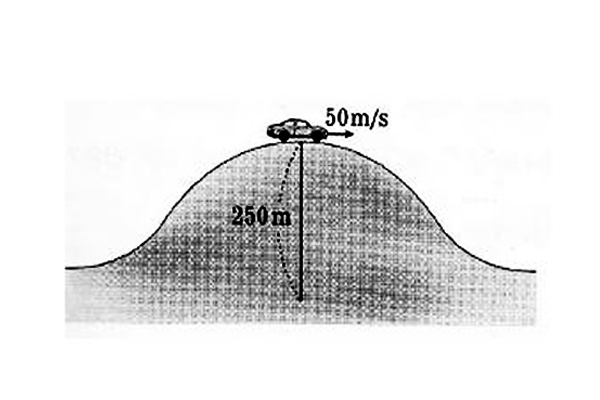# Normal forceThe above picture is a $1000$ kg car passing the top of a hill with radius of curvature $250$ m at a speed of $50$ m/s. Find the magnitude of normal force on the car (in N).

Gravitational acceleration is $g= 10$ m/s$^{2}$.

×

Problem Loading...

Note Loading...

Set Loading...